Share
Explore

#Summary FormulasSummary Formulas### How to do the equivalent of TABLE CALCULATORS in Coda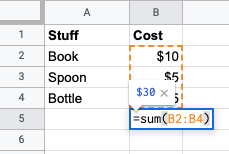Coda:
In Coda there are two ways of summarizing values from a table. You can have a Canvas Formula which will be separate from the table. And you can also have in table summary of the column.

Canvas Formula: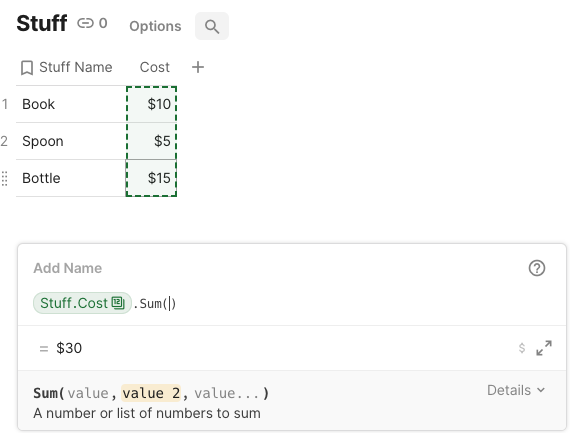Result: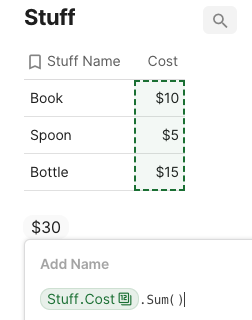In-table Summary: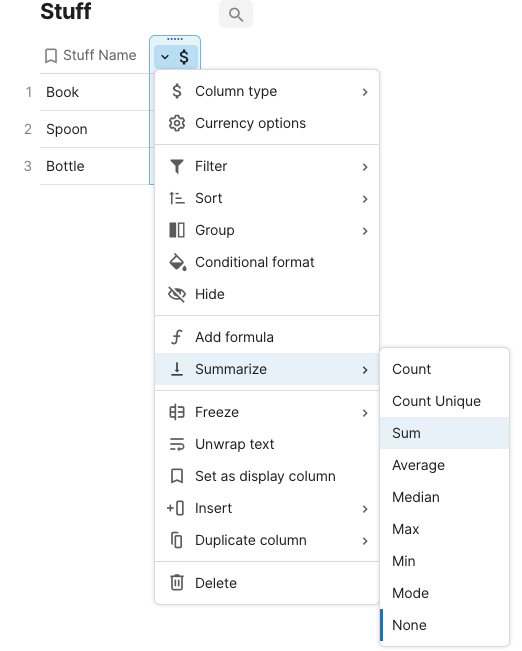Result: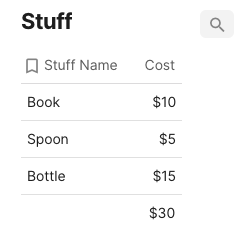### Formula side-by-side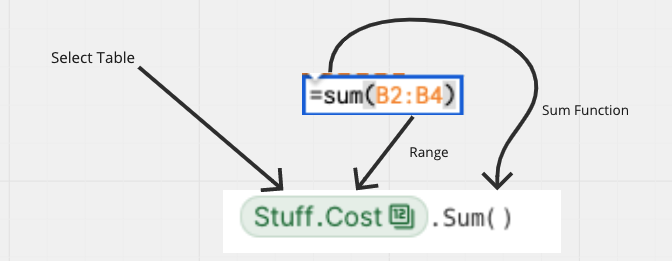Parameters
Summary in Excel
Summary in Coda
1
Dataset
Cell references (e.g. A2:B4)
Name of the table (e.g. “Stuff”)
2
Range to summarize
A range of values or a column (e.g. B2:B4 or “Cost”)
Name of the column you want to summarize (e.g. “Cost”)
3
Sum Function
A function to summarize the column or range (e.g. sum)
A function to summarize the column or range (e.g. sum)
There are no rows in this table### High-level differences between Summary Formulas and Canvas Formulas

Some of the main differences between Table Calculators in Google Sheets/Excel vs. Summary Formulas in Coda include:
Cell references vs names of tables/columns - Instead of having to reference a specific cell of a range of cells to reference a list of values, you can start a formula by typing the name of a table or column in the formula builder
Dots vs. parentheses - In addition to using parentheses in formulas, you can use dots as well. The dot “.” allows Coda to quickly auto-complete the name of the column you want to return from the lookup table. sum(table.column) is interchangeable with table.column.sum()
In table summary and canvas formula - In Coda, you have the ability to both summarize your list of values in the table you are referencing and use a canvas formula to summarize your range of values
Check out on Table Calculators in Excel/Google Sheets and Summary Formulas in Coda.